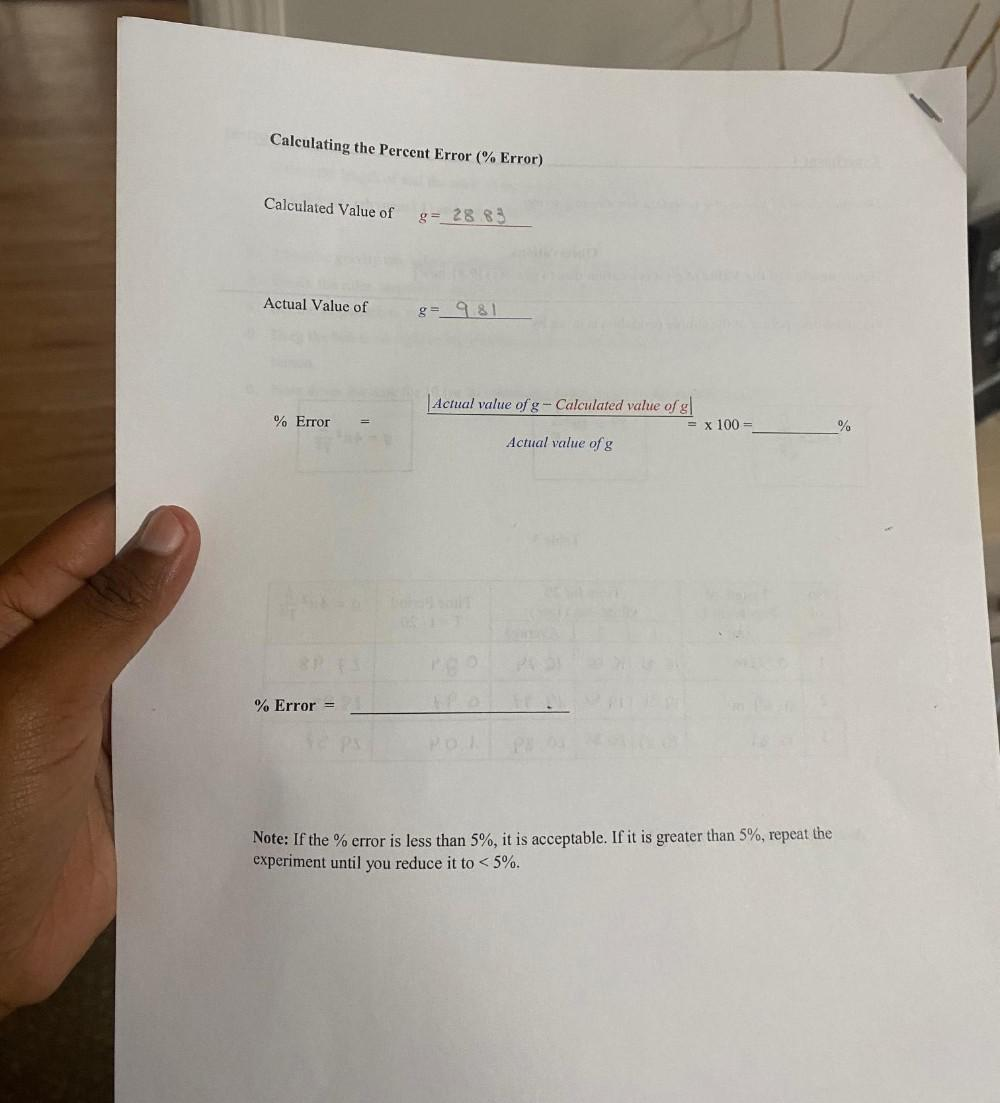Question:

# Calculating the Percent Error (% Error) Calculated Value of g= 28.83 Actual Value of g=_981 |Actual value of g- Calculated valueCalculating the Percent Error (% Error) Calculated Value of g= 28.83 Actual Value of g=_981 |Actual value of g- Calculated value of g| % Error = x 100 = % Actual value of g % Error = HO Note: If the % error is less than 5%, it is acceptable. If it is greater than 5%, repeat the experiment until you reduce it to < 5%.